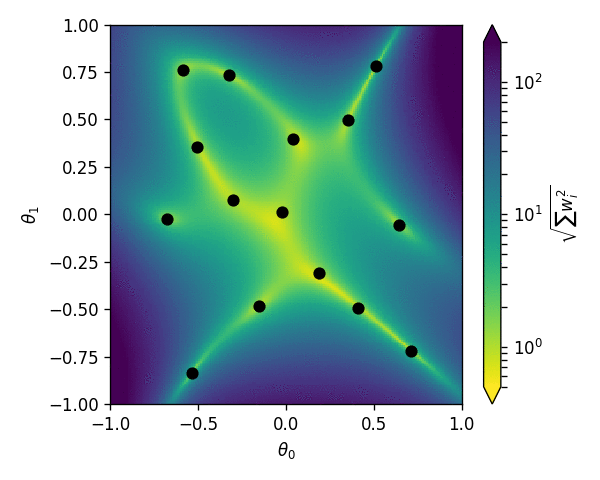# Interactive basis plaything¶

Allows you to interactively move the basis points (black dots) like in the animation below.import numpy as np
import bqplot
from bqplot import pyplot as plt
from IPython.display import display
from ipywidgets import Layout
import numpy as np
import pandas as pd
%matplotlib notebook
import sys

try:
from madminer.utils.morphing import PhysicsMorpher as Morpher
except ImportError:
sys.path.append('../..')
from madminer.utils.morphing import PhysicsMorpher as Morpher



## Settings¶

n_bases = 1
n_resolution = 50


## Preparation¶

morpher = Morpher(parameter_max_power=[4,4],
parameter_range=[(-1.,1.), (-1.,1.)])
_ = morpher.find_components(max_overall_power=4)

xi = np.linspace(-1.,1.,n_resolution)
yi = np.linspace(-1.,1.,n_resolution)
xx, yy = np.meshgrid(xi, yi)
xx = xx.reshape((-1,1))
yy = yy.reshape((-1,1))
theta_evaluation = np.hstack([xx, yy])

x_updated = False
y_updated = False


## Basis evaluation¶

def evaluate_basis(basis=None):

# Optimization
if basis is None:
basis = morpher.optimize_basis()

# Evaluate basis
squared_weights = []

for theta in theta_evaluation:
wi = morpher.calculate_morphing_weights(theta, basis)
squared_weights.append(np.sum(wi*wi)**0.5)

squared_weights = np.array(squared_weights).reshape((n_resolution,n_resolution))

return basis, squared_weights


## Initial set up¶

basis, squared_weights = evaluate_basis(None)


## Interactive tool¶

def update(change):
global basis, squared_weights, x_updated, y_updated

variable = change['name']
values = change['new']

if variable == 'x':
basis[:,0] = values
x_updated = True
elif variable == 'y':
basis[:,1] = values
y_updated = True

if x_updated and y_updated:
basis, squared_weights = evaluate_basis(basis)
heat.color = np.log(squared_weights) / np.log(10)

x_updated = False
y_updated = False

x_sc = bqplot.scales.LinearScale(min=-1., max=1.)
y_sc = bqplot.scales.LinearScale(min=-1., max=1.)
c_sc = bqplot.scales.ColorScale(min=0, max=1., scheme='YlOrRd')

heat = bqplot.GridHeatMap(color=np.log(squared_weights) / np.log(10),
scales={'row': x_sc, 'column': y_sc, 'color': c_sc},
row=yi,
column=xi,
stroke=None)

scatter = bqplot.Scatter(colors=['black']*len(basis[:,0]),
x=basis[:,0],
y=basis[:,1],
scales={'x': x_sc, 'y': y_sc})

ax_x = bqplot.Axis(scale=x_sc, label='fW')
ax_y = bqplot.Axis(scale=y_sc, orientation='vertical', label='fWW')
ax_c = bqplot.ColorAxis(scale=c_sc,
orientation='vertical',
side='right')

fig = bqplot.Figure(marks=[heat, scatter],
axes=[ax_x, ax_y, ax_c],
layout=Layout(width='600px', height='600px'))

display(fig)

scatter.observe(update, ['y','x'])
scatter.enable_move = True